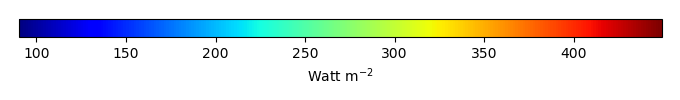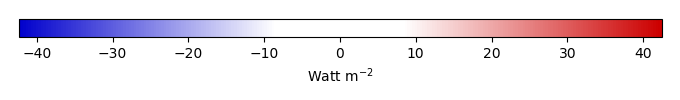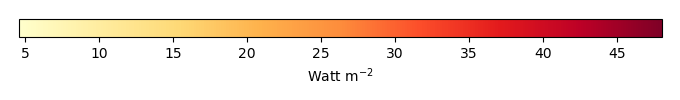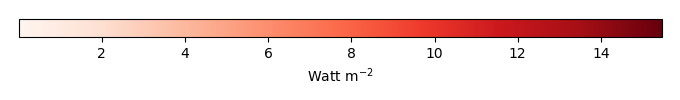# Mean State

Period Mean (original grids) [Watt m-2]
Model Period Mean (intersection) [Watt m-2]
Model Period Mean (complement) [Watt m-2]
Benchmark Period Mean (intersection) [Watt m-2]
Benchmark Period Mean (complement) [Watt m-2]
Bias [Watt m-2]
RMSE [Watt m-2]
Phase Shift [months]
Bias Score 
RMSE Score 
Seasonal Cycle Score 
Spatial Distribution Score 
Interannual Variability Score 
Overall Score 
Benchmark [-] 397.
CRUNCEPv7 [-] 406. 406. 396. 408. 11.3 16.6 0.567 0.327 0.403 0.940 0.763 0.761 0.599
GSWP3v1 [-] 395. 395. 396. 408. -0.219 6.93 0.212 0.821 0.497 0.974 0.999 0.754 0.757
WFDEI [-] 417. 418. 396. 408. 22.7 28.2 0.840 0.173 0.297 0.910 0.611 0.650 0.490
Period Mean (original grids) [Watt m-2]
Model Period Mean (intersection) [Watt m-2]
Model Period Mean (complement) [Watt m-2]
Benchmark Period Mean (intersection) [Watt m-2]
Benchmark Period Mean (complement) [Watt m-2]
Bias [Watt m-2]
RMSE [Watt m-2]
Phase Shift [months]
Bias Score 
RMSE Score 
Seasonal Cycle Score 
Spatial Distribution Score 
Interannual Variability Score 
Overall Score 
Benchmark [-] 384.
CRUNCEPv7 [-] 395. 394. 384. 397. 10.5 17.7 0.930 0.614 0.634 0.889 0.831 0.742 0.724
GSWP3v1 [-] 384. 384. 384. 397. -0.0965 9.48 0.181 0.904 0.688 0.985 0.999 0.910 0.862
WFDEI [-] 402. 402. 384. 397. 17.8 28.6 0.919 0.502 0.457 0.889 0.753 0.726 0.631
Period Mean (original grids) [Watt m-2]
Model Period Mean (intersection) [Watt m-2]
Model Period Mean (complement) [Watt m-2]
Benchmark Period Mean (intersection) [Watt m-2]
Benchmark Period Mean (complement) [Watt m-2]
Bias [Watt m-2]
RMSE [Watt m-2]
Phase Shift [months]
Bias Score 
RMSE Score 
Seasonal Cycle Score 
Spatial Distribution Score 
Interannual Variability Score 
Overall Score 
Benchmark [-] 362.
CRUNCEPv7 [-] 369. 368. 361. 382. 9.69 17.7 1.20 0.620 0.625 0.840 0.968 0.714 0.732
GSWP3v1 [-] 359. 359. 361. 382. 0.0495 8.96 0.237 0.879 0.702 0.971 1.00 0.852 0.851
WFDEI [-] 368. 368. 361. 382. 8.67 19.2 1.12 0.616 0.574 0.867 0.927 0.831 0.732
Period Mean (original grids) [Watt m-2]
Model Period Mean (intersection) [Watt m-2]
Model Period Mean (complement) [Watt m-2]
Benchmark Period Mean (intersection) [Watt m-2]
Benchmark Period Mean (complement) [Watt m-2]
Bias [Watt m-2]
RMSE [Watt m-2]
Phase Shift [months]
Bias Score 
RMSE Score 
Seasonal Cycle Score 
Spatial Distribution Score 
Interannual Variability Score 
Overall Score 
Benchmark [-] 239.
CRUNCEPv7 [-] 205. 205. 238. 251. -30.9 33.7 0.0673 0.550 0.783 0.995 0.970 0.740 0.803
GSWP3v1 [-] 236. 236. 238. 251. 0.254 9.46 0.0140 0.964 0.834 0.999 1.00 0.887 0.920
WFDEI [-] 206. 206. 238. 251. -30.0 34.6 0.0562 0.557 0.723 0.996 0.990 0.764 0.792
Period Mean (original grids) [Watt m-2]
Model Period Mean (intersection) [Watt m-2]
Model Period Mean (complement) [Watt m-2]
Benchmark Period Mean (intersection) [Watt m-2]
Benchmark Period Mean (complement) [Watt m-2]
Bias [Watt m-2]
RMSE [Watt m-2]
Phase Shift [months]
Bias Score 
RMSE Score 
Seasonal Cycle Score 
Spatial Distribution Score 
Interannual Variability Score 
Overall Score 
Benchmark [-] 411.
CRUNCEPv7 [-] 427. 427. 410. 415. 19.7 22.9 1.86 0.0696 0.331 0.721 0.566 0.701 0.453
GSWP3v1 [-] 408. 408. 410. 415. 0.0344 5.44 0.531 0.668 0.397 0.922 0.993 0.736 0.686
WFDEI [-] 438. 438. 410. 415. 28.7 30.2 1.77 0.0477 0.223 0.733 0.724 0.586 0.423
Period Mean (original grids) [Watt m-2]
Model Period Mean (intersection) [Watt m-2]
Model Period Mean (complement) [Watt m-2]
Benchmark Period Mean (intersection) [Watt m-2]
Benchmark Period Mean (complement) [Watt m-2]
Bias [Watt m-2]
RMSE [Watt m-2]
Phase Shift [months]
Bias Score 
RMSE Score 
Seasonal Cycle Score 
Spatial Distribution Score 
Interannual Variability Score 
Overall Score 
Benchmark [-] 264.
CRUNCEPv7 [-] 243. 243. 264. 277. -20.2 22.6 0.0306 0.696 0.838 0.998 0.983 0.858 0.869
GSWP3v1 [-] 264. 263. 264. 277. 0.0194 8.45 0.00704 0.975 0.861 1.00 1.00 0.811 0.918
WFDEI [-] 243. 243. 264. 277. -20.9 25.9 0.0255 0.687 0.763 0.998 0.993 0.875 0.846
Period Mean (original grids) [Watt m-2]
Model Period Mean (intersection) [Watt m-2]
Model Period Mean (complement) [Watt m-2]
Benchmark Period Mean (intersection) [Watt m-2]
Benchmark Period Mean (complement) [Watt m-2]
Bias [Watt m-2]
RMSE [Watt m-2]
Phase Shift [months]
Bias Score 
RMSE Score 
Seasonal Cycle Score 
Spatial Distribution Score 
Interannual Variability Score 
Overall Score 
Benchmark [-] 294.
CRUNCEPv7 [-] 279. 278. 293. 336. -11.9 19.7 0.0697 0.761 0.775 0.995 0.992 0.827 0.854
GSWP3v1 [-] 291. 291. 293. 336. 0.277 9.99 0.0242 0.939 0.825 0.998 1.00 0.784 0.895
WFDEI [-] 280. 279. 293. 336. -10.8 21.6 0.101 0.759 0.731 0.993 0.996 0.836 0.841
Period Mean (original grids) [Watt m-2]
Model Period Mean (intersection) [Watt m-2]
Model Period Mean (complement) [Watt m-2]
Benchmark Period Mean (intersection) [Watt m-2]
Benchmark Period Mean (complement) [Watt m-2]
Bias [Watt m-2]
RMSE [Watt m-2]
Phase Shift [months]
Bias Score 
RMSE Score 
Seasonal Cycle Score 
Spatial Distribution Score 
Interannual Variability Score 
Overall Score 
Benchmark [-] 379.
CRUNCEPv7 [-] 385. 385. 377. 410. 14.7 23.2 0.641 0.526 0.667 0.941 0.933 0.740 0.746
GSWP3v1 [-] 371. 372. 377. 410. -0.454 9.29 0.100 0.882 0.746 0.991 1.00 0.877 0.874
WFDEI [-] 390. 391. 377. 410. 19.8 27.6 0.682 0.481 0.587 0.931 0.956 0.807 0.725
Period Mean (original grids) [Watt m-2]
Model Period Mean (intersection) [Watt m-2]
Model Period Mean (complement) [Watt m-2]
Benchmark Period Mean (intersection) [Watt m-2]
Benchmark Period Mean (complement) [Watt m-2]
Bias [Watt m-2]
RMSE [Watt m-2]
Phase Shift [months]
Bias Score 
RMSE Score 
Seasonal Cycle Score 
Spatial Distribution Score 
Interannual Variability Score 
Overall Score 
Benchmark [-] 244.
CRUNCEPv7 [-] 214. 215. 244. 248. -28.8 31.6 0.00253 0.558 0.773 1.00 0.972 0.838 0.819
GSWP3v1 [-] 243. 243. 244. 248. 0.0310 8.41 0.00100 0.978 0.842 1.00 1.00 0.883 0.924
WFDEI [-] 215. 215. 244. 248. -28.2 33.6 0.00539 0.564 0.691 1.00 0.990 0.877 0.802
Period Mean (original grids) [Watt m-2]
Model Period Mean (intersection) [Watt m-2]
Model Period Mean (complement) [Watt m-2]
Benchmark Period Mean (intersection) [Watt m-2]
Benchmark Period Mean (complement) [Watt m-2]
Bias [Watt m-2]
RMSE [Watt m-2]
Phase Shift [months]
Bias Score 
RMSE Score 
Seasonal Cycle Score 
Spatial Distribution Score 
Interannual Variability Score 
Overall Score 
Benchmark [-] 351.
CRUNCEPv7 [-] 353. 353. 350. 359. 3.02 14.9 0.381 0.746 0.720 0.974 0.875 0.714 0.792
GSWP3v1 [-] 350. 350. 350. 359. 0.0571 7.85 0.104 0.931 0.779 0.993 1.00 0.909 0.899
WFDEI [-] 352. 352. 350. 359. 1.80 17.8 0.496 0.765 0.621 0.965 0.897 0.838 0.785
Period Mean (original grids) [Watt m-2]
Model Period Mean (intersection) [Watt m-2]
Model Period Mean (complement) [Watt m-2]
Benchmark Period Mean (intersection) [Watt m-2]
Benchmark Period Mean (complement) [Watt m-2]
Bias [Watt m-2]
RMSE [Watt m-2]
Phase Shift [months]
Bias Score 
RMSE Score 
Seasonal Cycle Score 
Spatial Distribution Score 
Interannual Variability Score 
Overall Score 
Benchmark [-] 308.
CRUNCEPv7 [-] 290. 290. 307. 324. -13.0 18.2 0.220 0.649 0.738 0.985 0.897 0.856 0.810
GSWP3v1 [-] 304. 304. 307. 324. 0.0546 8.06 0.0712 0.930 0.782 0.995 1.00 0.739 0.871
WFDEI [-] 288. 288. 307. 324. -15.4 22.3 0.317 0.612 0.643 0.979 0.903 0.900 0.780
Period Mean (original grids) [Watt m-2]
Model Period Mean (intersection) [Watt m-2]
Model Period Mean (complement) [Watt m-2]
Benchmark Period Mean (intersection) [Watt m-2]
Benchmark Period Mean (complement) [Watt m-2]
Bias [Watt m-2]
RMSE [Watt m-2]
Phase Shift [months]
Bias Score 
RMSE Score 
Seasonal Cycle Score 
Spatial Distribution Score 
Interannual Variability Score 
Overall Score 
Benchmark [-] 344.
CRUNCEPv7 [-] 305. 305. 311. 360. -2.78 21.3 0.550 0.591 0.634 0.933 0.991 0.728 0.752
GSWP3v1 [-] 308. 308. 311. 360. -0.0963 9.16 0.113 0.894 0.716 0.987 1.00 0.838 0.858
WFDEI [-] 306. 306. 311. 360. -2.14 24.0 0.579 0.581 0.575 0.930 0.994 0.776 0.739
Period Mean (original grids) [Watt m-2]
Model Period Mean (intersection) [Watt m-2]
Model Period Mean (complement) [Watt m-2]
Benchmark Period Mean (intersection) [Watt m-2]
Benchmark Period Mean (complement) [Watt m-2]
Bias [Watt m-2]
RMSE [Watt m-2]
Phase Shift [months]
Bias Score 
RMSE Score 
Seasonal Cycle Score 
Spatial Distribution Score 
Interannual Variability Score 
Overall Score 
Benchmark [-] 343.
CRUNCEPv7 [-] 350. 350. 343. 360. 8.96 20.6 0.463 0.703 0.633 0.956 0.933 0.670 0.755
GSWP3v1 [-] 342. 342. 343. 360. 0.000187 11.3 0.0949 0.917 0.723 0.990 1.00 0.894 0.874
WFDEI [-] 339. 339. 343. 360. -1.65 19.2 0.513 0.795 0.605 0.953 0.999 0.710 0.778
Period Mean (original grids) [Watt m-2]
Model Period Mean (intersection) [Watt m-2]
Model Period Mean (complement) [Watt m-2]
Benchmark Period Mean (intersection) [Watt m-2]
Benchmark Period Mean (complement) [Watt m-2]
Bias [Watt m-2]
RMSE [Watt m-2]
Phase Shift [months]
Bias Score 
RMSE Score 
Seasonal Cycle Score 
Spatial Distribution Score 
Interannual Variability Score 
Overall Score 
Benchmark [-] 244.
CRUNCEPv7 [-] 216. 216. 243. 253. -27.1 29.3 0.0689 0.559 0.791 0.995 0.989 0.754 0.813
GSWP3v1 [-] 243. 243. 243. 253. -0.0103 8.60 0.0247 0.965 0.833 0.998 1.00 0.881 0.919
WFDEI [-] 216. 216. 243. 253. -27.5 31.6 0.0782 0.554 0.724 0.995 0.981 0.834 0.802
Period Mean (original grids) [Watt m-2]
Model Period Mean (intersection) [Watt m-2]
Model Period Mean (complement) [Watt m-2]
Benchmark Period Mean (intersection) [Watt m-2]
Benchmark Period Mean (complement) [Watt m-2]
Bias [Watt m-2]
RMSE [Watt m-2]
Phase Shift [months]
Bias Score 
RMSE Score 
Seasonal Cycle Score 
Spatial Distribution Score 
Interannual Variability Score 
Overall Score 
Benchmark [-] 374.
CRUNCEPv7 [-] 372. 372. 369. 398. 13.3 21.8 0.734 0.451 0.593 0.917 0.934 0.773 0.710
GSWP3v1 [-] 362. 362. 369. 398. 0.0604 9.12 0.254 0.816 0.684 0.968 1.00 0.867 0.837
WFDEI [-] 376. 376. 369. 398. 15.8 25.2 0.633 0.432 0.524 0.934 0.951 0.796 0.693
Period Mean (original grids) [Watt m-2]
Model Period Mean (intersection) [Watt m-2]
Model Period Mean (complement) [Watt m-2]
Benchmark Period Mean (intersection) [Watt m-2]
Benchmark Period Mean (complement) [Watt m-2]
Bias [Watt m-2]
RMSE [Watt m-2]
Phase Shift [months]
Bias Score 
RMSE Score 
Seasonal Cycle Score 
Spatial Distribution Score 
Interannual Variability Score 
Overall Score 
Benchmark [-] 332.
CRUNCEPv7 [-] 321. 321. 331. 370. -6.68 20.0 0.0934 0.788 0.682 0.994 0.912 0.890 0.824
GSWP3v1 [-] 328. 328. 331. 370. -0.0972 7.51 0.0275 0.951 0.841 0.998 1.00 0.841 0.912
WFDEI [-] 319. 319. 331. 370. -8.96 23.1 0.127 0.783 0.627 0.991 0.933 0.829 0.799
Period Mean (original grids) [Watt m-2]
Model Period Mean (intersection) [Watt m-2]
Model Period Mean (complement) [Watt m-2]
Benchmark Period Mean (intersection) [Watt m-2]
Benchmark Period Mean (complement) [Watt m-2]
Bias [Watt m-2]
RMSE [Watt m-2]
Phase Shift [months]
Bias Score 
RMSE Score 
Seasonal Cycle Score 
Spatial Distribution Score 
Interannual Variability Score 
Overall Score 
Benchmark [-] 277.
CRUNCEPv7 [-] 254. 254. 276. 298. -20.7 23.2 0.0749 0.619 0.793 0.995 0.995 0.906 0.850
GSWP3v1 [-] 274. 275. 276. 298. -0.0815 7.66 0.0260 0.950 0.841 0.998 1.00 0.828 0.910
WFDEI [-] 253. 254. 276. 298. -21.3 26.3 0.0831 0.614 0.704 0.994 0.996 0.866 0.813
Period Mean (original grids) [Watt m-2]
Model Period Mean (intersection) [Watt m-2]
Model Period Mean (complement) [Watt m-2]
Benchmark Period Mean (intersection) [Watt m-2]
Benchmark Period Mean (complement) [Watt m-2]
Bias [Watt m-2]
RMSE [Watt m-2]
Phase Shift [months]
Bias Score 
RMSE Score 
Seasonal Cycle Score 
Spatial Distribution Score 
Interannual Variability Score 
Overall Score 
Benchmark [-] 296.
CRUNCEPv7 [-] 286. 287. 295. 324. -7.01 15.9 0.214 0.747 0.729 0.986 0.942 0.838 0.828
GSWP3v1 [-] 293. 294. 295. 324. -0.178 9.51 0.0536 0.911 0.770 0.996 1.00 0.791 0.873
WFDEI [-] 287. 289. 295. 324. -6.04 17.7 0.241 0.768 0.664 0.983 0.970 0.817 0.811
Period Mean (original grids) [Watt m-2]
Model Period Mean (intersection) [Watt m-2]
Model Period Mean (complement) [Watt m-2]
Benchmark Period Mean (intersection) [Watt m-2]
Benchmark Period Mean (complement) [Watt m-2]
Bias [Watt m-2]
RMSE [Watt m-2]
Phase Shift [months]
Bias Score 
RMSE Score 
Seasonal Cycle Score 
Spatial Distribution Score 
Interannual Variability Score 
Overall Score 
Benchmark [-] 406.
CRUNCEPv7 [-] 424. 424. 405. 410. 18.6 23.4 2.09 0.149 0.368 0.665 0.721 0.746 0.503
GSWP3v1 [-] 405. 405. 405. 410. -0.270 6.26 0.342 0.796 0.499 0.953 1.00 0.824 0.762
WFDEI [-] 432. 432. 405. 410. 27.3 31.7 2.65 0.0848 0.296 0.562 0.725 0.535 0.417
Period Mean (original grids) [Watt m-2]
Model Period Mean (intersection) [Watt m-2]
Model Period Mean (complement) [Watt m-2]
Benchmark Period Mean (intersection) [Watt m-2]
Benchmark Period Mean (complement) [Watt m-2]
Bias [Watt m-2]
RMSE [Watt m-2]
Phase Shift [months]
Bias Score 
RMSE Score 
Seasonal Cycle Score 
Spatial Distribution Score 
Interannual Variability Score 
Overall Score 
Benchmark [-] 376.
CRUNCEPv7 [-] 384. 383. 376. 374. 8.34 16.3 1.28 0.586 0.603 0.806 0.908 0.839 0.724
GSWP3v1 [-] 376. 375. 376. 374. -0.175 9.06 0.144 0.889 0.661 0.985 1.00 0.837 0.839
WFDEI [-] 386. 385. 376. 374. 9.58 19.0 1.13 0.594 0.515 0.852 0.934 0.834 0.707
Period Mean (original grids) [Watt m-2]
Model Period Mean (intersection) [Watt m-2]
Model Period Mean (complement) [Watt m-2]
Benchmark Period Mean (intersection) [Watt m-2]
Benchmark Period Mean (complement) [Watt m-2]
Bias [Watt m-2]
RMSE [Watt m-2]
Phase Shift [months]
Bias Score 
RMSE Score 
Seasonal Cycle Score 
Spatial Distribution Score 
Interannual Variability Score 
Overall Score 
Benchmark [-] 307.
CRUNCEPv7 [-] 289. 288. 303. 340. -11.5 22.3 0.489 0.460 0.572 0.957 0.987 0.700 0.708
GSWP3v1 [-] 301. 301. 303. 340. 1.13 13.5 0.185 0.721 0.579 0.981 0.998 0.747 0.768
WFDEI [-] 298. 297. 303. 340. -3.55 21.7 0.545 0.512 0.509 0.954 0.986 0.763 0.706

# Temporally integrated period mean

BENCHMARK MEANMODEL MEANBIASBIAS SCORERMSERMSE SCOREBENCHMARK INTERANNUAL VARIABILITYMODEL INTERANNUAL VARIABILITYINTERANNUAL VARIABILITY SCOREBENCHMARK MAX MONTHMODEL MAX MONTHDIFFERENCE IN MAX MONTHSEASONAL CYCLE SCORESPATIAL TAYLOR DIAGRAMMODEL COLORS# Spatially integrated regional mean

MODEL COLORSREGIONAL MEANANNUAL CYCLEMONTHLY ANOMALYANNUAL CYCLE# All Models

BenchmarkCRUNCEPv7GSWP3v1WFDEI# Data Information

creation_date: Mon Jun 30 23:41:07 PDT 2014

source_file: This product is generated from monthly 1 degree GEWEX SRB Radiation observations